## Wednesday, March 30, 2016

### Changes to `plotSimmap` (and depend functions) for left-facing trees

I just posted an important update in the way that `direction="leftwards"` trees are plotted using `plotSimmap`, `plotTree`, or any other phytools function that uses `plotSimmap` internally (and there are many such functions).

The change is that in prior versions of `plotSimmap` `direction="leftwards"` was accomplished just by flipping the direction of the x-axis of the plot. Now instead the direcction is flipped by first flipping the coordinate system of the plotted tree, and then plotting it on unfliped axes!

This will (hopefully) be of little consequence to most users. Please let me know ASAP if any plotting functions of phytools (or other packages that import phytools) are detrimentally affected.

Note that it is still possible to flip the x axis if desired.

Here is a demo:

``````library(phytools)
packageVersion("phytools")
``````
``````##  '0.5.25'
``````
``````tree
``````
``````##
## Phylogenetic tree with 41 tips and 40 internal nodes.
##
## Tip labels:
##  t1, t2, t3, t35, t36, t24, ...
##
## Rooted; includes branch lengths.
``````
``````plotTree(tree,ftype="off",direction="leftwards",
mar=c(5.1,1.1,1.1,1.1))
axis(1)
``````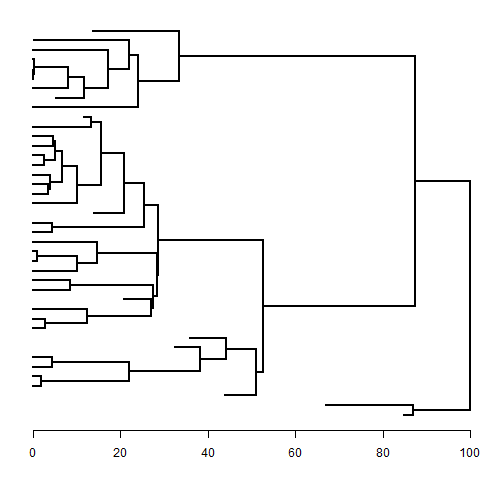Note that in prior versions of phytools, the axis would have been increasing from right to left by default with `direction="leftwards"`, as follows:

``````plotTree(tree,ftype="off",xlim=c(100,0),
mar=c(5.1,1.1,1.1,1.1))
axis(1)
``````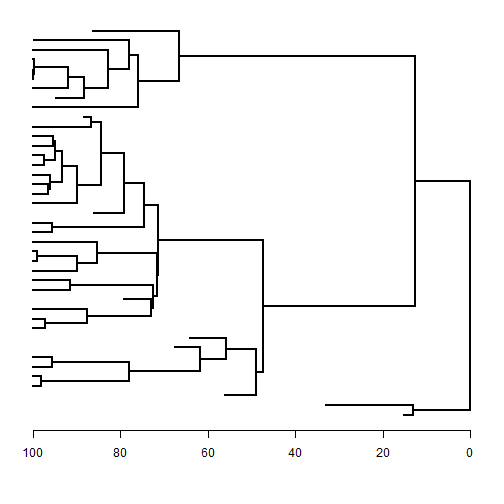The preceding is actually a `direction="rightwards"` tree facing left!

We can also use `direction="leftwards"` combined with a flipped x axis to get a right facing tree in which the axis shows time before present:

``````plotTree(tree,ftype="off",mar=c(5.1,1.1,1.1,1.1),xlim=c(100,0),
direction="leftwards")
axis(1)
title(xlab="time before present")
``````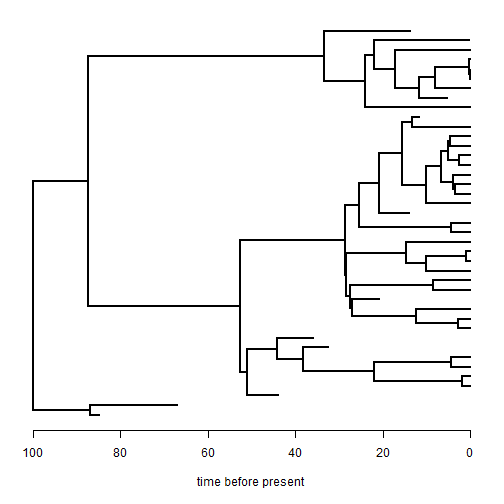The reason I started working on this was because I was encountering some weird behavior of ape's function `nodelabels` with trees plotted in a leftward direction. For instance, for the phytools `plot` method for objects of class `"describe.simmap"`:

``````tree
``````
``````##
## Phylogenetic tree with 41 tips and 40 internal nodes.
##
## Tip labels:
##  t1, t2, t3, t35, t36, t24, ...
##
## Rooted; includes branch lengths.
``````
``````x
``````
``````##  t1  t2  t3 t35 t36 t24 t25  t5  t4 t31 t32 t15  t7 t19 t20 t17 t37 t38
##   a   a   a   a   a   a   b   a   b   b   b   b   a   b   b   a   a   a
## t12 t26 t27 t10 t18 t29 t30 t28 t33 t34 t22 t23 t13 t14  t8 t16 t21 t40
##   a   a   a   a   a   a   a   a   a   b   a   a   a   a   b   b   b   b
## t41 t39 t11  t9  t6
##   b   b   b   b   a
## Levels: a b
``````
``````trees<-make.simmap(tree,x,nsim=100)
``````
``````## make.simmap is sampling character histories conditioned on the transition matrix
##
## Q =
##             a           b
## a -0.01531859  0.01531859
## b  0.01531859 -0.01531859
## (estimated using likelihood);
## and (mean) root node prior probabilities
## pi =
##   a   b
## 0.5 0.5
``````
``````## Done.
``````
``````obj<-summary(trees)
plot(obj) ## works fine
``````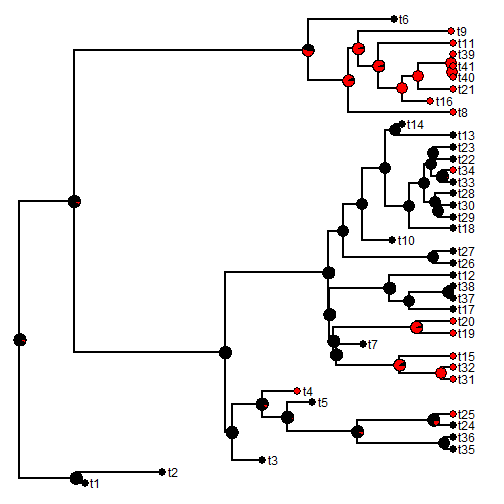``````plot(obj,direction="leftwards") ## did not work
``````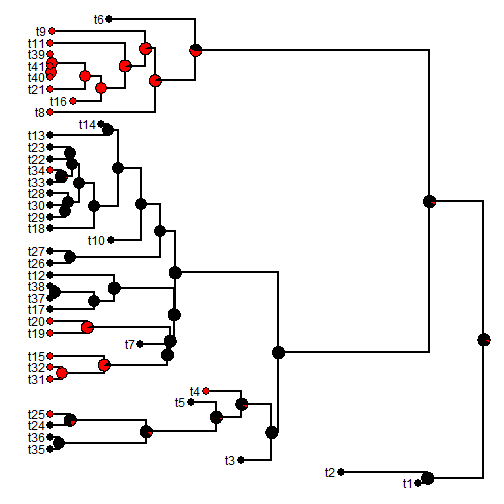I'm not sure why, but I'm guessing that this had to do with flipping x instead of the coordinate system of the tree when plotting leftwards because when we simulate this, the problem indeed reappears:

``````plot(obj,xlim=c(105,-12))
``````
``````## Error in symbols(xpos, ypos, circles = radius, inches = FALSE, add = TRUE, : invalid symbol parameter
``````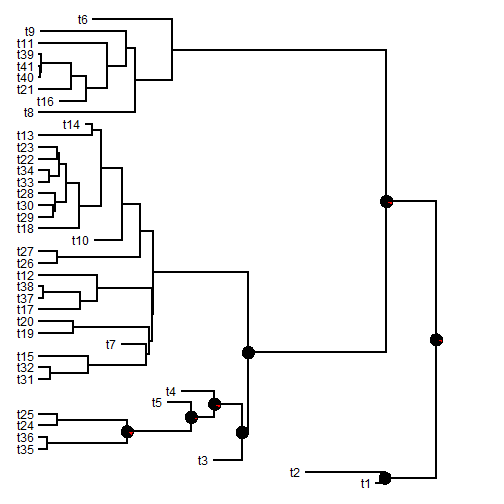As noted this could affect other phytools functions that use `plotSimmap` internally. For instance, it is easy to show that `plot.contMap` (for instance) is affected. E.g.:

``````y
``````
``````##      t1      t2      t3     t35     t36     t24     t25      t5      t4
##  0.2632 -0.4295 -2.0453 -3.3031 -2.7865 -3.5570 -0.5442 -2.9101  2.3353
##     t31     t32     t15      t7     t19     t20     t17     t37     t38
##  6.1904  6.2993  8.6808  7.1557  9.9159  6.8316 -1.6050 -0.7766 -2.1087
##     t12     t26     t27     t10     t18     t29     t30     t28     t33
##  4.5222  7.6534 10.2747  3.6215 12.9971  9.8071  8.2382  9.9934  9.9321
##     t34     t22     t23     t13     t14      t8     t16     t21     t40
##  8.7258  7.1663  6.8482  8.4406  8.0642  5.0276  9.1044  9.7583  9.2502
##     t41     t39     t11      t9      t6
##  9.0959  8.9720  9.6105  3.3538  3.0264
``````
``````obj<-contMap(tree,y) ## plots fine
``````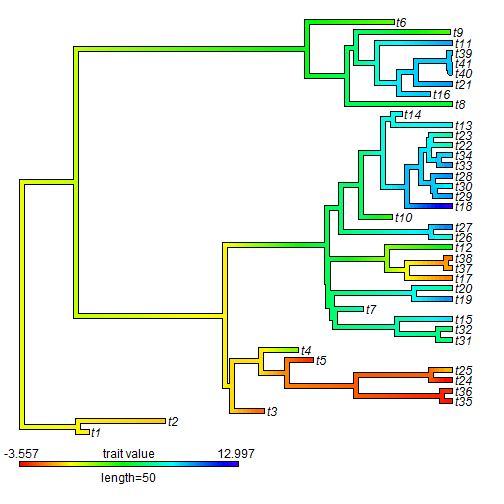``````plot(obj,direction="leftwards") ## legend is backwards!
``````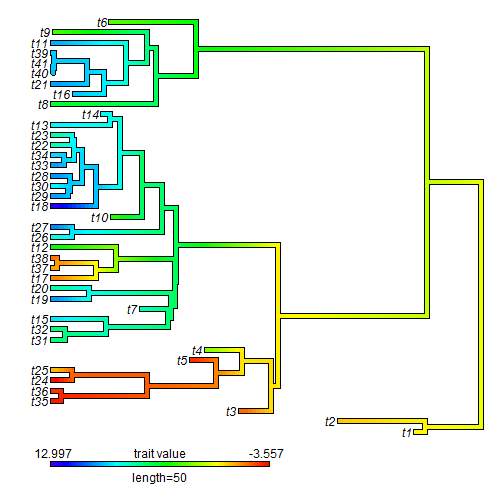I can fix this (and will shortly), however please alert me to any other similar issues that you encounter. Thanks!

Data for this exercise were simulated as follows:

``````tree<-pbtree(n=30,b=1,d=0.4,scale=100)
Q<-matrix(c(-1,1,1,-1),2,2)/100
rownames(Q)<-colnames(Q)<-letters[1:2]
x<-as.factor(sim.history(tree,Q)\$states)
y<-round(fastBM(tree),4)
``````

The latest phytools version can be installed from GitHub as follows:

``````library(devtools)
install_github("liamrevell/phytools")
packageVersion("phytools")
``````

## Tuesday, March 29, 2016

### `as.phylo.formula` in which a given taxonomic level is found at a constant depth

Unfortunately, the R-sig-phylo archive was recently made private due to some issues with spammers. This means that the links in several prior phytools blog posts may be dead. Sorry! If you encounter this issue and it is affecting your research, please let me know. I can try to update the link or supply information on how to find the archived messages on the searchable archive, which is not private.

The one post that I didn't realize was popular until the archive went down was a very simple modification that I made to the ape function `as.phylo.formula` that I described in a phytools blog post here. In this modification, divergences of a certain taxonomic level (e.g., family) are all forced to have the same depth.

For the record, here is the code:

``````## as.phylo.formula from ape written by Julien Dutheil
## (julien.duth...@univ-montp2.fr)
## modified by Liam Revell (liam.rev...@umb.edu)
## depends on ape & phytools
as.phylo.formula<-function (x, data = parent.frame(), ...){
err<-"Formula must be of the kind \"~A1/A2/.../An\"."
if(length(x)!=2)
stop(err)
if(x[]!="~")
stop(err)
f<-x[]
taxo<-list()
while(length(f)==3){
if(f[]!="/")
stop(err)
if(!is.factor(data[[deparse(f[])]]))
stop(paste("Variable",deparse(f[]),
"must be a factor."))
taxo[[deparse(f[])]]<-data[[deparse(f[])]]
if(length(f)>1)
f<-f[]
}
if(!is.factor(data[[deparse(f)]]))
stop(paste("Variable",deparse(f),"must be a factor."))
taxo[[deparse(f)]]<-data[[deparse(f)]]
taxo.data<-as.data.frame(taxo)
leaves.names<-as.character(taxo.data[,1])
taxo.data[,1]<-1:nrow(taxo.data)
f.rec<-function(subtaxo){
u<-ncol(subtaxo)
levels<-unique(subtaxo[,u])
if(u==1){
if(length(levels)!=nrow(subtaxo))
warning("Error, leaves names are not unique.")
return(as.character(subtaxo[,1]))
}
t<-character(length(levels))
for(l in 1:length(levels)){
x<-f.rec(subtaxo[subtaxo[,u]==levels[l],][1:(u-1)])
t[l]<-paste("(",paste(x,collapse=","),")",sep="")
}
return(t)
}
string<-paste("(",paste(f.rec(taxo.data),collapse=","),
");",sep="")
## so that singles will be read without error
phy\$edge.length<-rep(1,nrow(phy\$edge))
phy<-collapse.singles(phy)
phy\$tip.label<-leaves.names[as.numeric(phy\$tip.label)]
return(phy)
}
``````

And here's a demo of it's use:

``````library(phytools)
data(carnivora)
t1<-as.phylo.formula(~SuperFamily/Family/Genus/Species,
data=carnivora)
plotTree(t1,fsize=0.7,ftype="i")
``````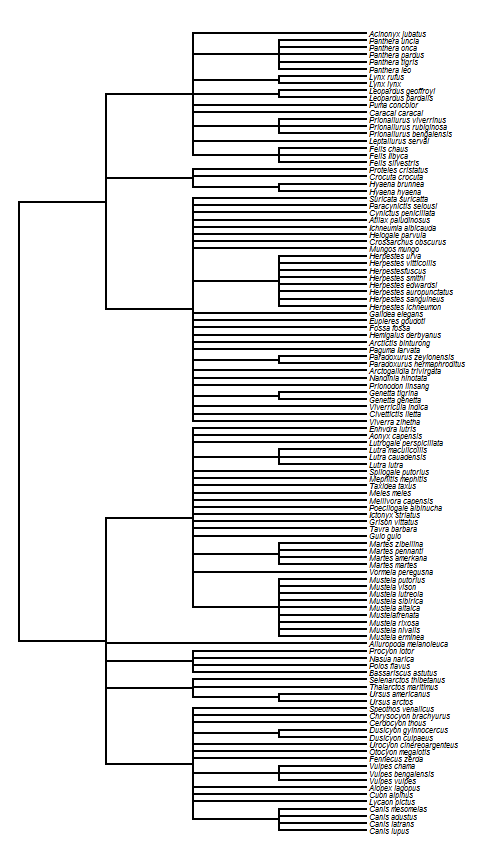You can try it with & without loading the modified function version, above, to see the difference.